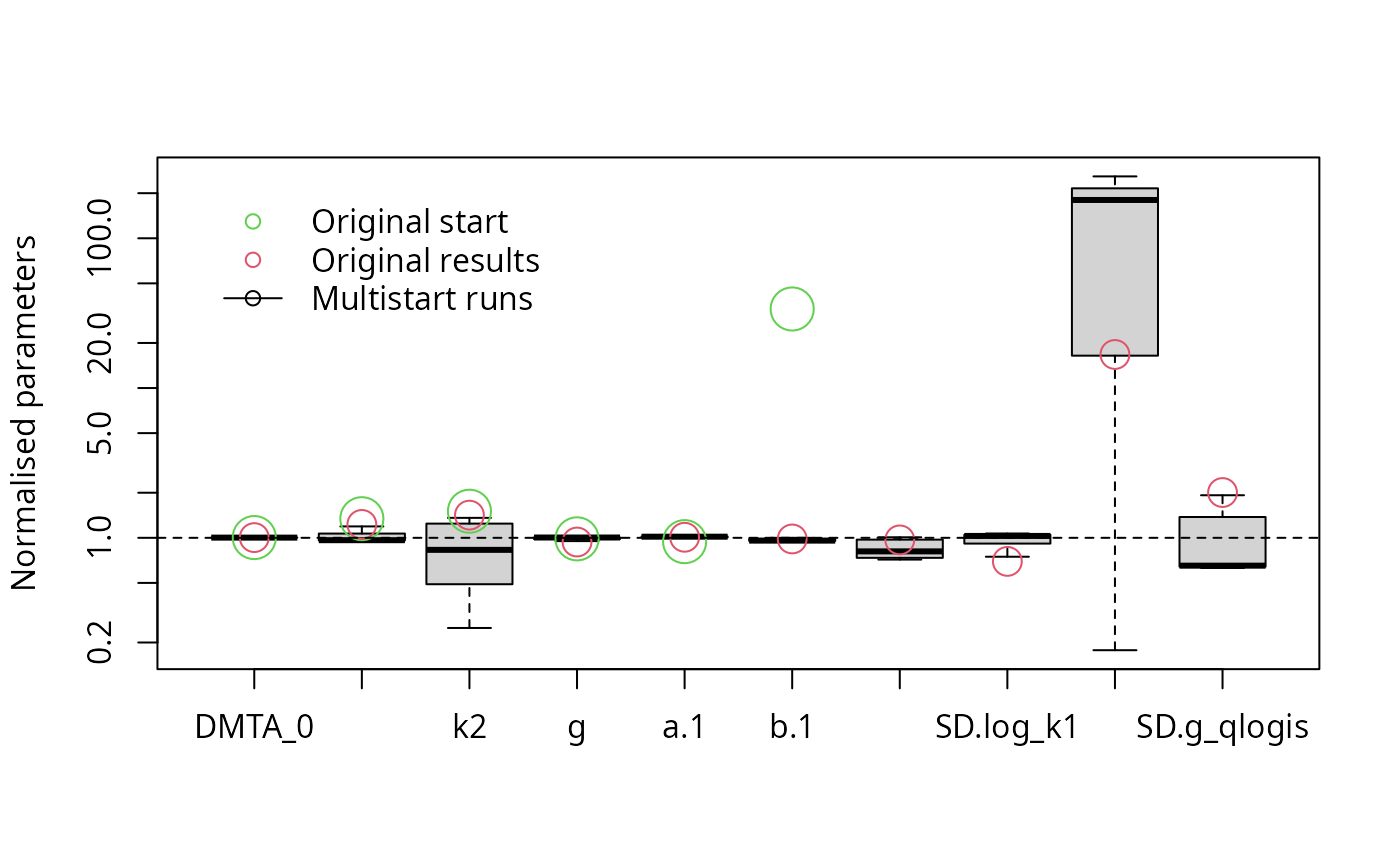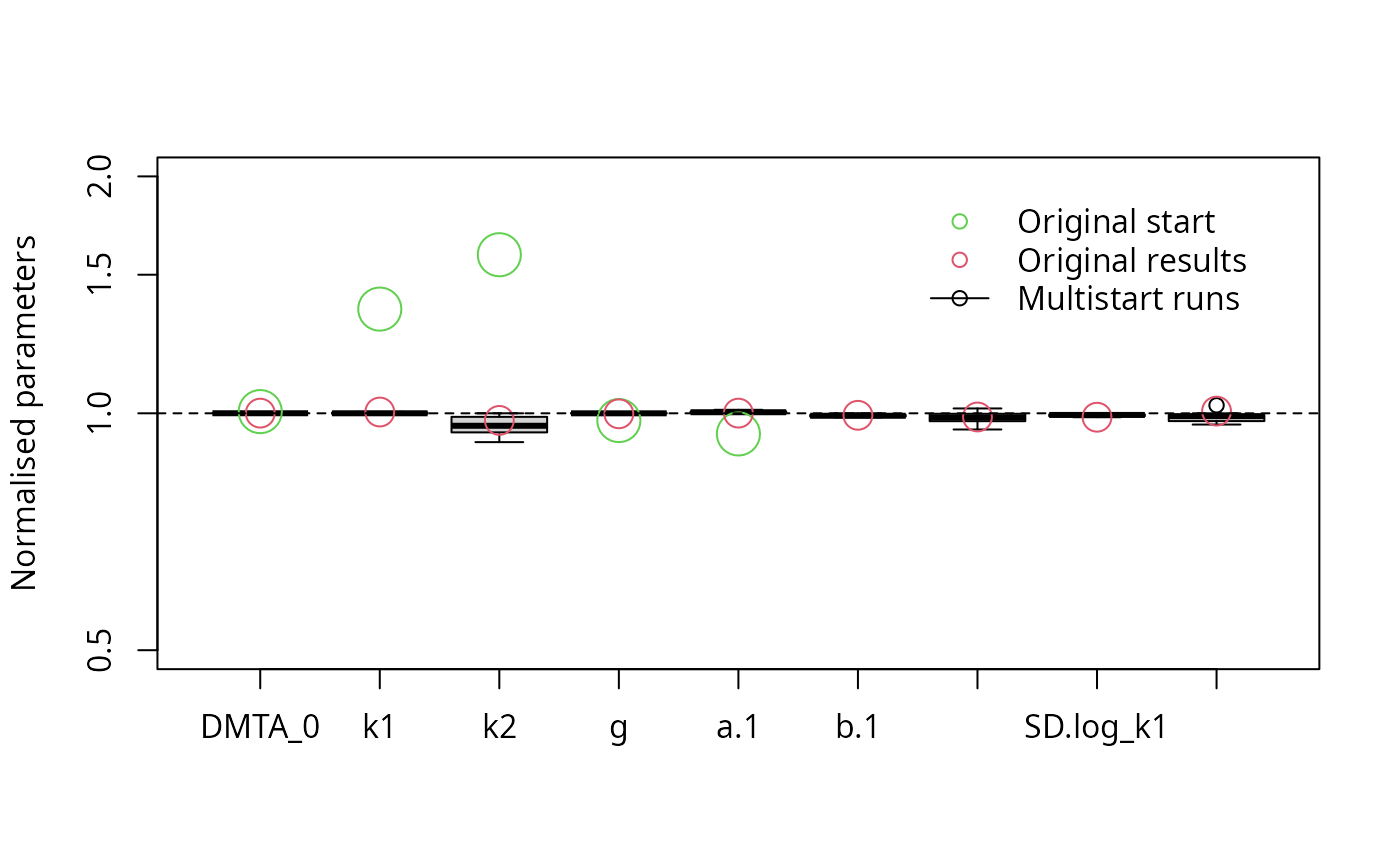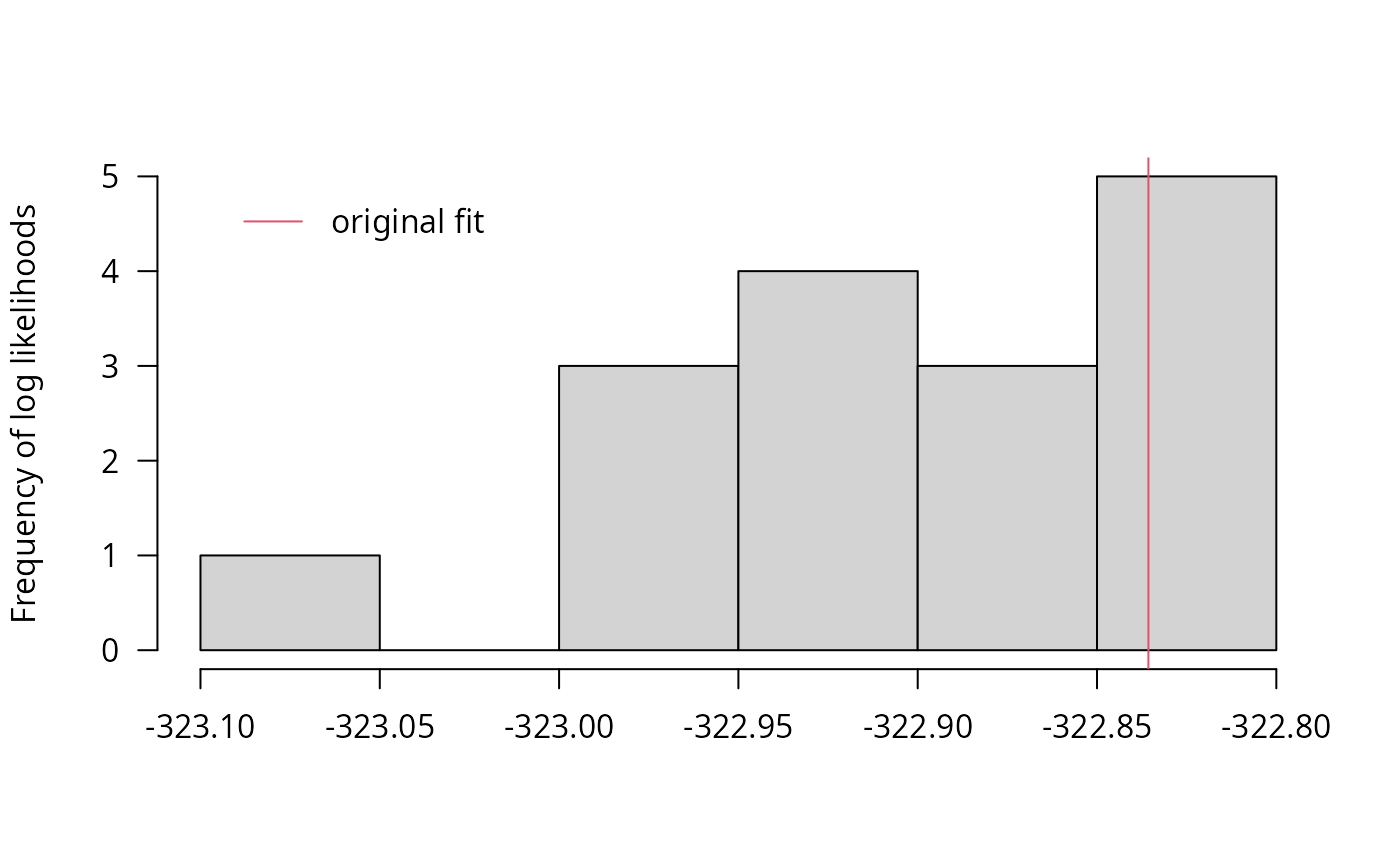The dimethenamid data from 2018 from seven soils is used as example data in this vignette.

library(mkin)
dmta_ds <- lapply(1:7, function(i) {
ds_i <- dimethenamid_2018$ds[[i]]$data
ds_i[ds_i$name == "DMTAP", "name"] <- "DMTA" ds_i$time <- ds_i$time * dimethenamid_2018$f_time_norm[i]
ds_i
})
names(dmta_ds) <- sapply(dimethenamid_2018$ds, function(ds) ds$title)
dmta_ds[["Elliot"]] <- rbind(dmta_ds[["Elliot 1"]], dmta_ds[["Elliot 2"]])
dmta_ds[["Elliot 1"]] <- dmta_ds[["Elliot 2"]] <- NULL

First, we check the DFOP model with the two-component error model and random effects for all degradation parameters.

f_mmkin <- mmkin("DFOP", dmta_ds, error_model = "tc", cores = 7, quiet = TRUE)
f_saem_full <- saem(f_mmkin)
illparms(f_saem_full)
##  "sd(log_k2)"

We see that not all variability parameters are identifiable. The illparms function tells us that the confidence interval for the standard deviation of ‘log_k2’ includes zero. We check this assessment using multiple runs with different starting values.

f_saem_full_multi <- multistart(f_saem_full, n = 16, cores = 16)
parplot(f_saem_full_multi)This confirms that the variance of k2 is the most problematic parameter, so we reduce the parameter distribution model by removing the intersoil variability for k2.

f_saem_reduced <- update(f_saem_full, no_random_effect = "log_k2")
illparms(f_saem_reduced)
f_saem_reduced_multi <- multistart(f_saem_reduced, n = 16, cores = 16)
parplot(f_saem_reduced_multi, lpos = "topright")The results confirm that all remaining parameters can be determined with sufficient certainty.

We can also analyse the log-likelihoods obtained in the multiple runs:

llhist(f_saem_reduced_multi)The parameter histograms can be further improved by excluding the result with the low likelihood.

parplot(f_saem_reduced_multi, lpos = "topright", llmin = -326, ylim = c(0.5, 2))We can use the anova method to compare the models, including a likelihood ratio test if the models are nested.

anova(f_saem_full, best(f_saem_reduced_multi), test = TRUE)
## Data: 155 observations of 1 variable(s) grouped in 6 datasets
##
##                            npar    AIC    BIC     Lik Chisq Df Pr(>Chisq)
## best(f_saem_reduced_multi)    9 663.69 661.82 -322.85
## f_saem_full                  10 669.77 667.69 -324.89     0  1          1

While AIC and BIC are lower for the reduced model, the likelihood ratio test does not indicate a significant difference between the fits.